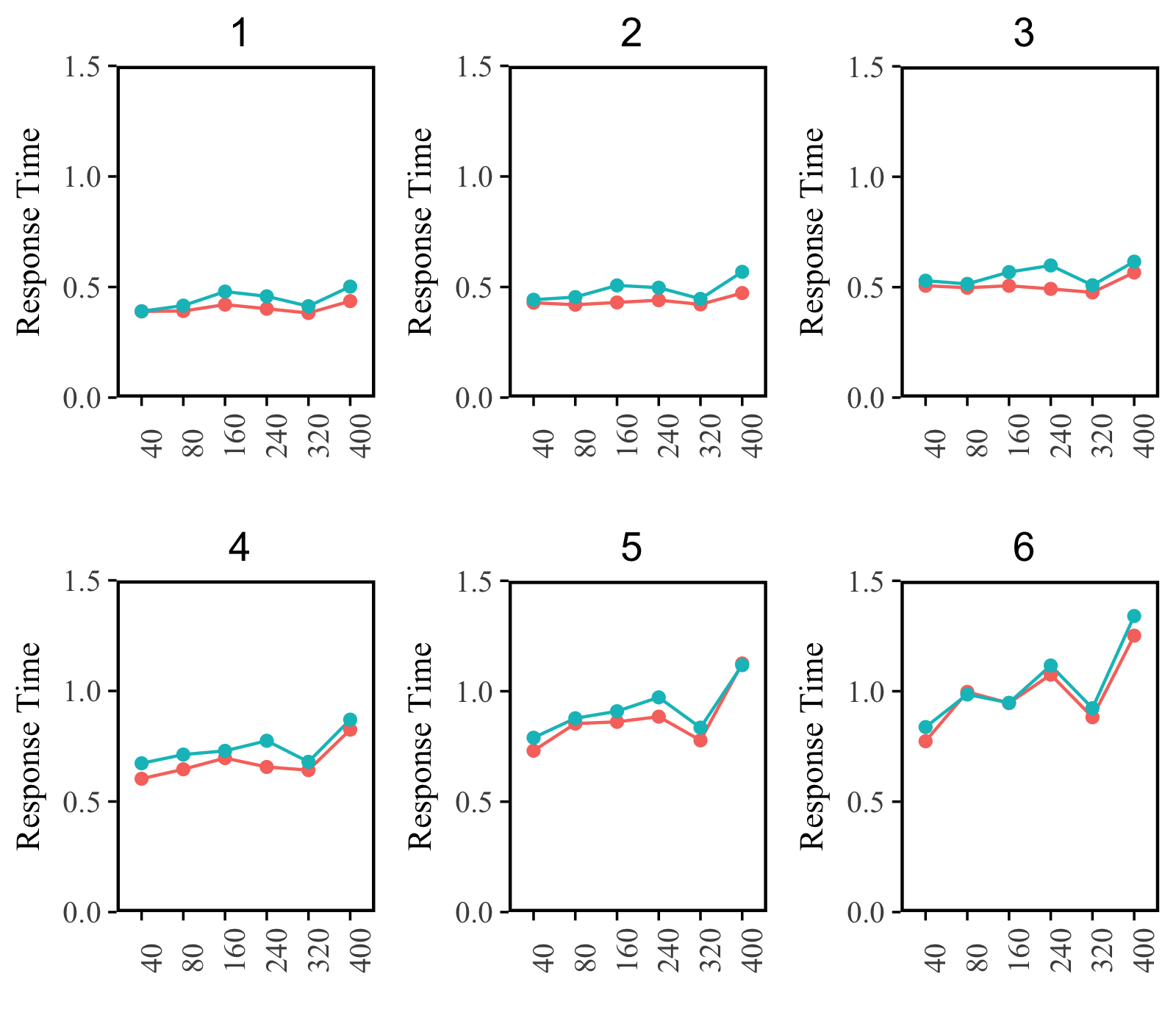# Plot multiple panels in one figure with ggplot2

ggplot2 is versatile but sometimes it also has limits. One example is that ggplot2 has difficulty in ploting multiple panels into one figure (like the built-in function par() ).

A solution for this comes from the “Cookbook for R”, in which provides a home made function “multiplot”. This function works with ggplot2 and can plot multiple panels into one single figure.

Users only need to plot figures using ggplot2 as usual, and at the end of the plotting, put all those figures together with the function multiplot.

For convenience, I paste the function in this blog, you may also go the original page to read more.

Here is an exmaple figure I draw based on this function.